# RD Sharma Solutions Class 12 The Plane Exercise 29.4

RD Sharma Solutions for Class 12 Maths Chapter 29 The Plane Exercise 29.4, is provided here to help students score good marks in the exam. In order to enhance the performance of students in the Class 12 exam, we at BYJU’S have formulated the RD Sharma Solutions for Class 12 Maths. Students can easily download the pdf consisting of this chapter solutions, which are available in the links provided below and can use it for future reference as well.

## Download PDF of RD Sharma Solutions For Class 12 Maths Chapter 29 Exercise 4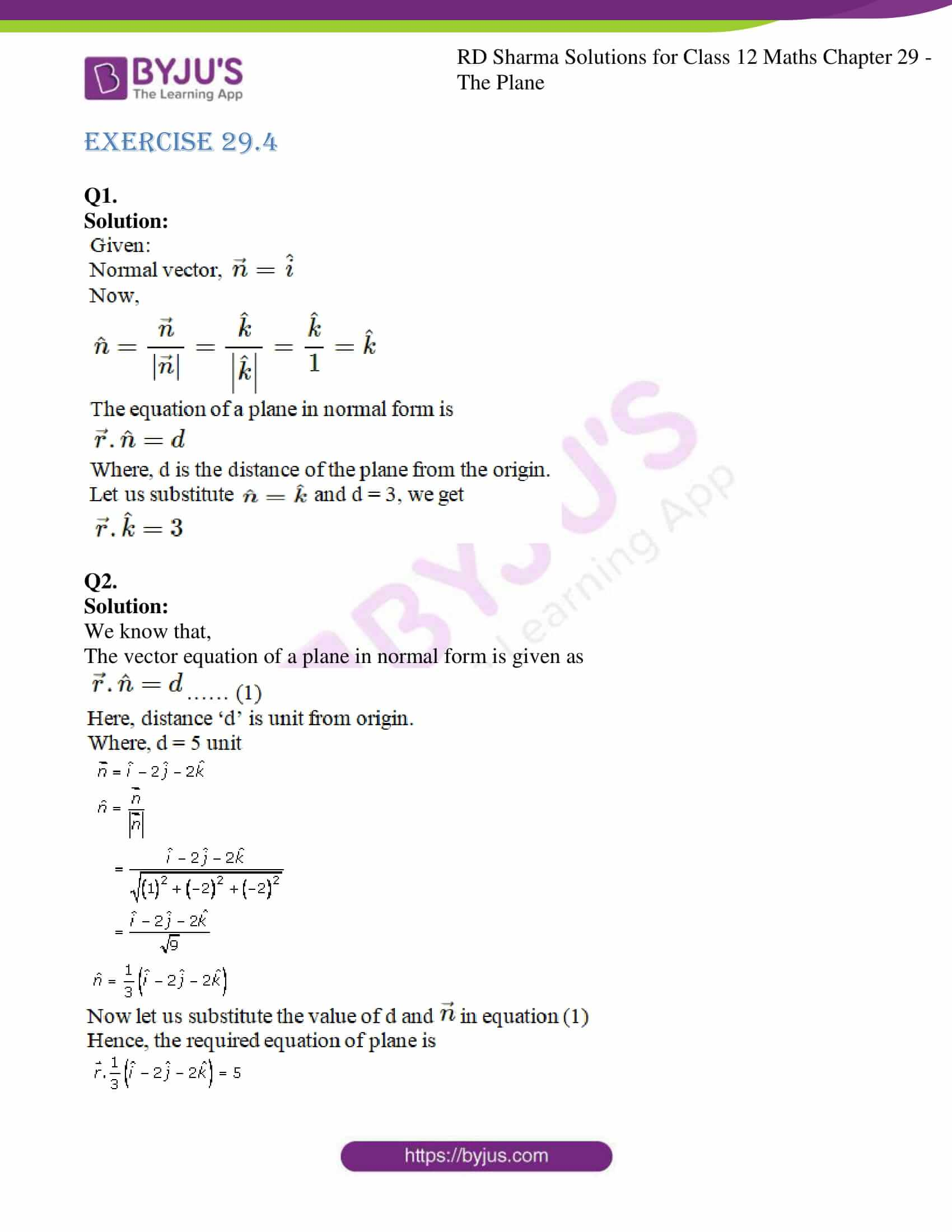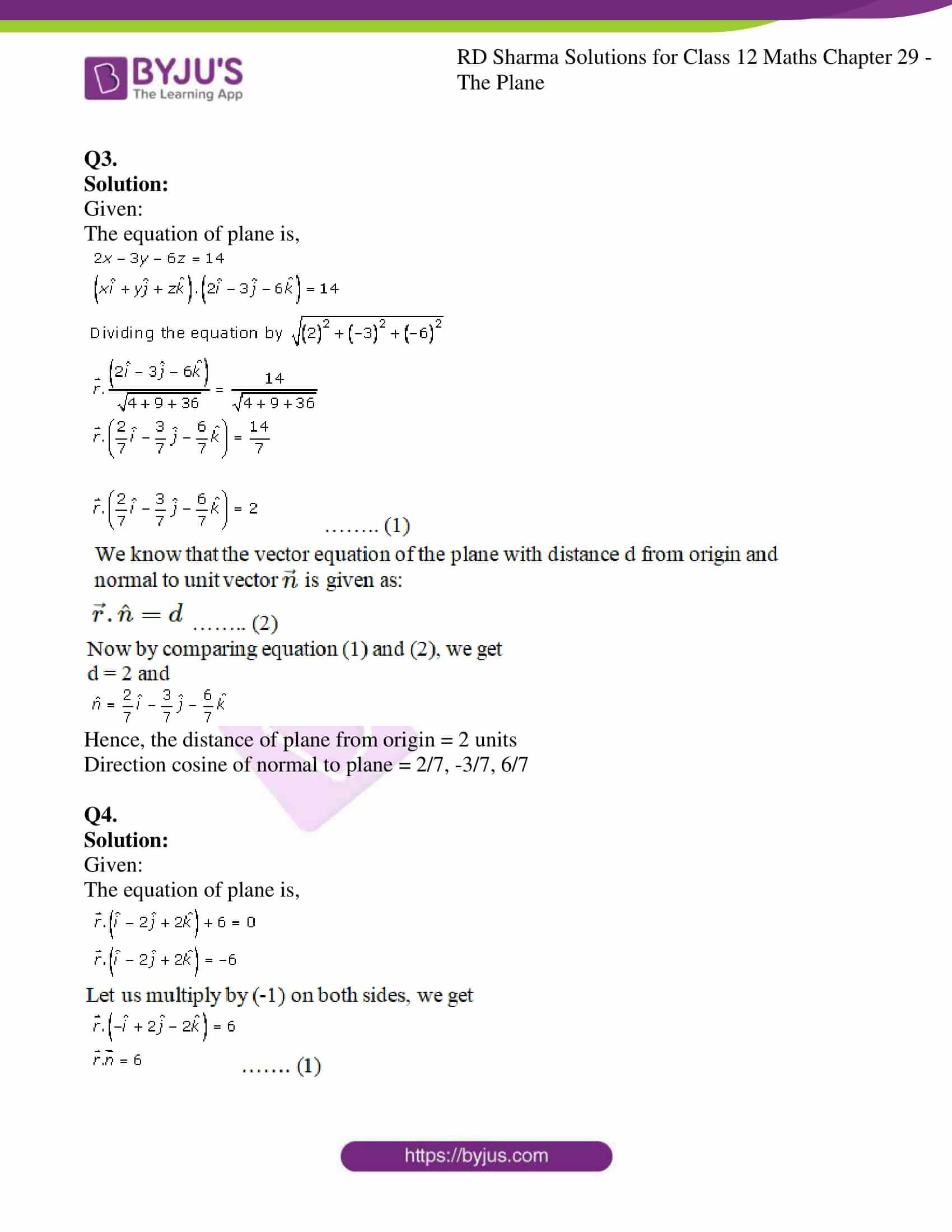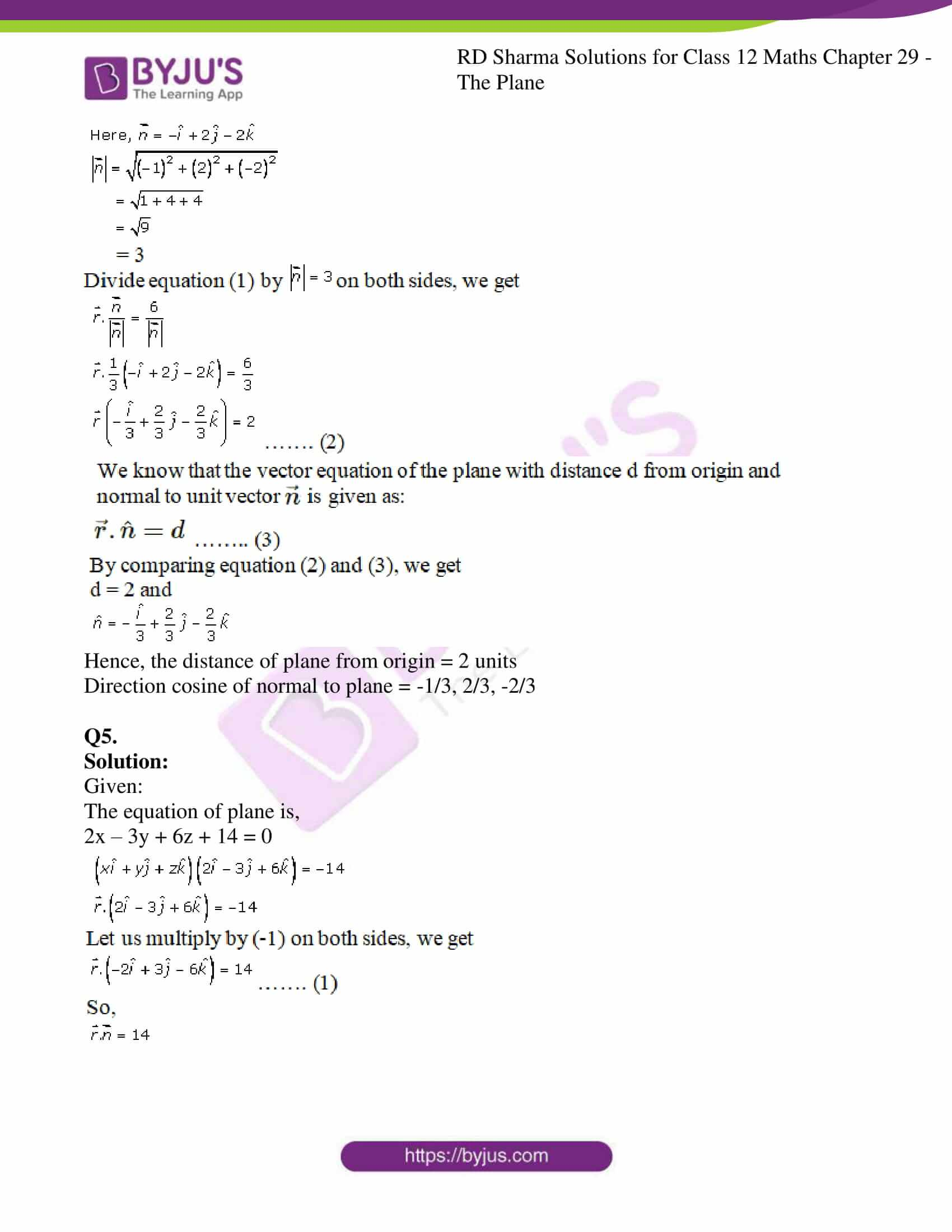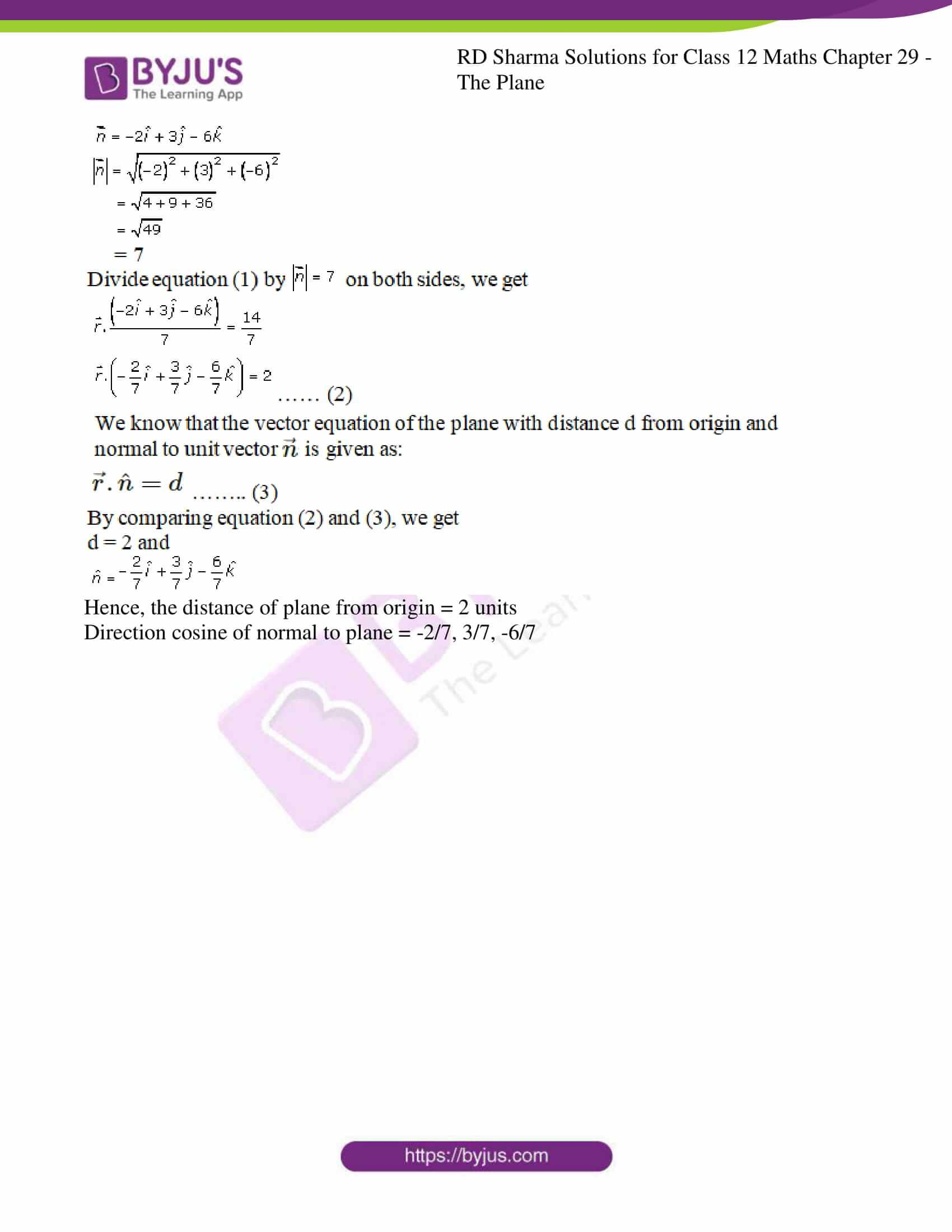### Access RD Sharma Solutions For Class 12 Maths Chapter 29 Exercise 4

EXERCISE 29.4

Q1.

Solution: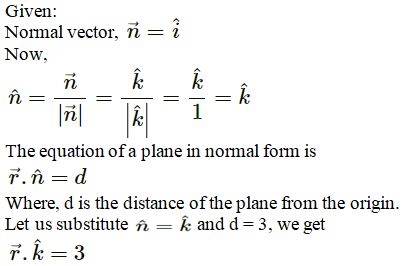Q2.

Solution:

We know that,

The vector equation of a plane in normal form is given as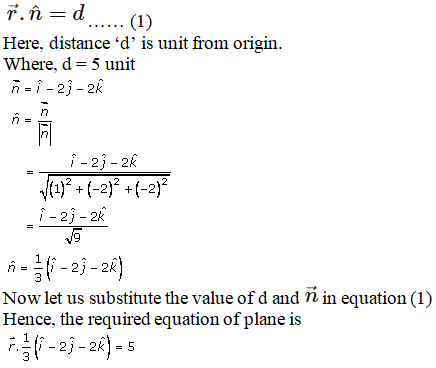Q3.

Solution:

Given:

The equation of plane is,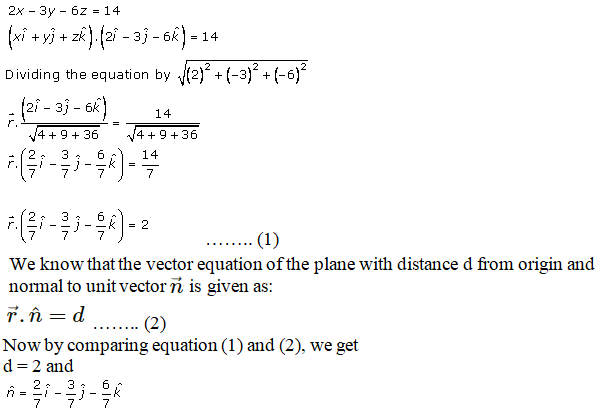Hence, the distance of plane from origin = 2 units

Direction cosine of normal to plane = 2/7, -3/7, 6/7

Q4.

Solution:

Given:

The equation of plane is,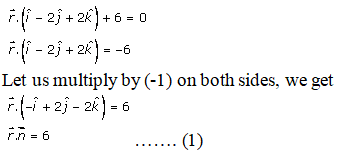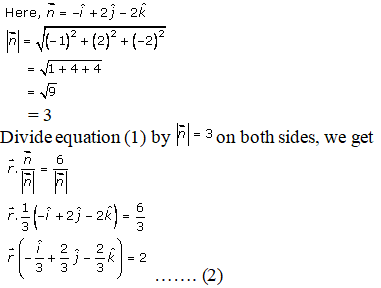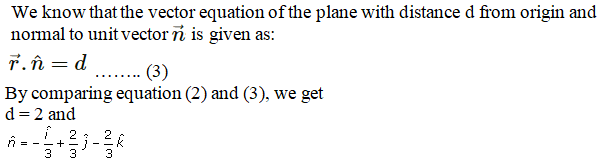Hence, the distance of plane from origin = 2 units

Direction cosine of normal to plane = -1/3, 2/3, -2/3

Q5.

Solution:

Given:

The equation of plane is,

2x – 3y + 6z + 14 = 0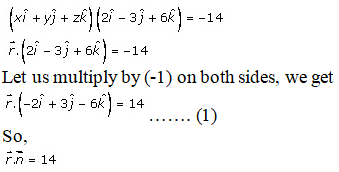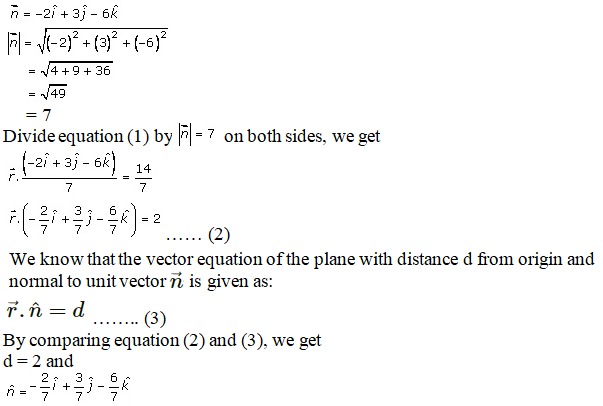Hence, the distance of plane from origin = 2 units

Direction cosine of normal to plane = -2/7, 3/7, -6/7